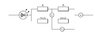# Determine the ratio of resistance

• TyErd

## Homework Statement

Determine the value of the ratio R1/R2

sorry guys I forgot to write the values in, V1=0, V2=2.4V and A=10.8A

V=IR

## The Attempt at a Solution

I found thecurrent through R2 to be 6mA and then calculated resistance by doing 2.4/6mA=400ohms. Then i got stuck.

#### Attachments

•circuit8.JPG
9.7 KB · Views: 388
Last edited:

How could you possibly have calculated 6 mA unless you know V1, V2, and A? I think the problem is asking for R1/R2 in terms of V1, V2, and A.

The only way we could assume the potential drop across V1 and V2 is to know the colour of the LED...but we don't have that.

If you have the equation R=V/I then you can form equations for each resistor R1 and R2; if you are aiming for higher grades (>=A) at college level you should know how to form ratios of equations.

Sorry guys I forgot to add the values in. My mistake. I've added the values in just then.